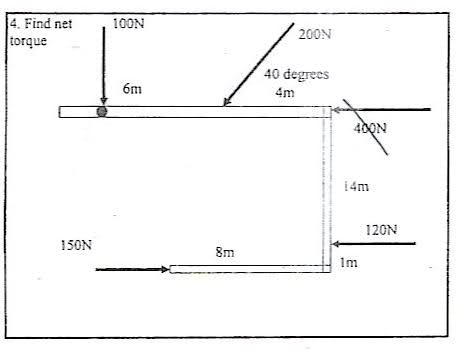# Torque Problem

## Homework Statement

Find net torque.## Homework Equations

T = F(d) and T = F(d)sinx

Torque = T
Force = F
d = distance

## The Attempt at a Solution

100(0) - 200(4)sin40 - 120(14) + 150(8) = -994.2 Nm

Did I do this correctly? Notice I crossed out the 400N also because I think that it does not affect it.

LowlyPion
Homework Helper
Welcome to PF.

I think your second term is 6m from the pivot not 4m.

Your bottom force - 150N - is 15m from the pivot not 8m as your equation suggests.

Ah, thanks, but how is 150N 15m away from the pivot point? Do I just go up? Sorry I do not understand :(

LowlyPion
Homework Helper
Ah, thanks, but how is 150N 15m away from the pivot point? Do I just go up? Sorry I do not understand :(

It's the projection of the force to a line that is perpendicular to the pivot. That is the way you should be taking Torque.

For instance the one at an angle of 40° if you extended that out you would see that the lever arm that is perpendicular to the force is Sin40° times the 6m length.

For the bottom force it is acting horizontally, but it is acting through the vertical line from the pivot at a distance of 14 + 1 = 15.

Ahhh...that clears up so many things. Thank you so much!